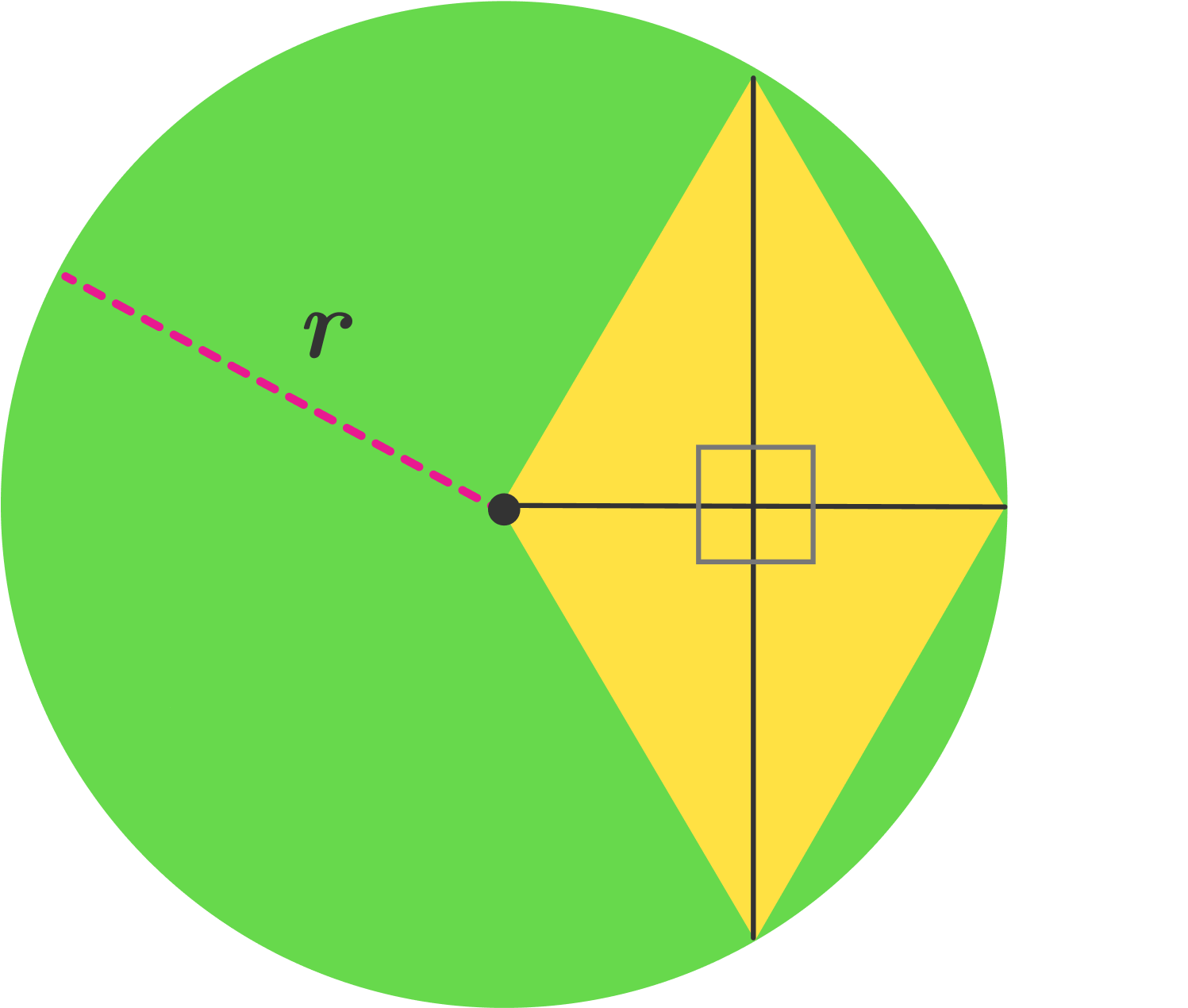# A Rhombus Gets Cozy In A Circle

Geometry Level 1A rhombus is inscribed in a circle with radius $r$ and center $O$, such that one of the vertices lies on $O$ and rest lie on the circumference of the circle.

Find the area of the rhombus in terms of $r$.

×